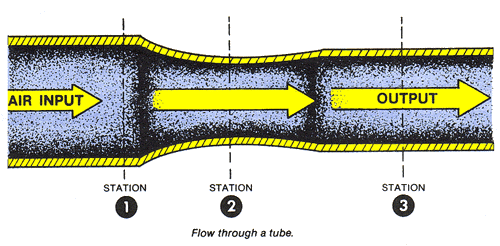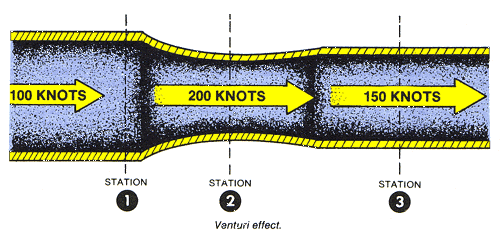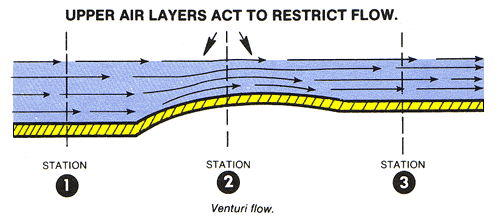Aerodynamics concerns the motion of air and other gaseous fluids and other forces acting on objects in motion through the air (gases). In effect, Aerodynamics is concerned with the object (aircraft), the movement (Relative Wind), and the air (Atmosphere).

Newton's Laws Of Motion

Newton's three laws of motion are:Inertia - A body at rest will remain at rest. and a body in motion will remain in motion at the same speed and direction until affected by some external force. Nothing starts or stops without an outside force to bring about or prevent motion. Hence, the force with which a body offers resistance to change is called the force of inertia.Acceleration - The force required to produce a change in motion of a body is directly proportional to its mass and the rate of change in its velocity. Acceleration refers either to an increase or a decrease in velocity, although Deceleration is commonly used to indicate a decrease.Action / Reaction - For every action there is an equal and opposite reaction. If an interaction occurs between two bodies, equal forces in opposite directions will be imparted to each body.

Fluid Flow And Airspeed Measurement (Bernoulli's Principle)

Daniel Bernoulli, a Swiss mathematician, stated a principle that describes the relationship between internal fluid pressure and fluid velocity. His principle, essentially a statement of the conversation of energy, explains at least in part why an airfoil develops an aerodynamic force.

All of the forces acting on a surface over which there is a flow of air are the result of skin friction or pressure. Friction forces are the result of viscosity and are confined to a very thin layer of air near the surface. They usually are not dominant and, from the aviator's perspective, can be discounted.As an aid in visualizing what happens to pressure as air flows over an airfoil, it is helpful to consider flow through a tube (Please see Figure above). The concept of conservation of mass states that mass cannot be created or destroyed; so, what goes in one end of the tube must come out the other end. If the flow through a tube is neither acccelerating or decelerating at the input, then the mass of flow per unit of time at Station 1 must equal the mass of flow per unit of time at Station 2, and so on, through Station 3. The mass of flow per unit area (cross-sectional area of tube) is called the Mass Flow Rate.

At low flight speeds, air experiences relatively small changes in pressure and negligable changes in density. This airflow is termed incompressable since the air may undergo changes in pressure without apparent changes in density. Such airflow is similar to the flow of water, hydraulic fluid, or any other incompressable fluid. This suggests that between any two points in the tube, the velocity varies inversely with the area. Venturi effect is the name used to describe this phenomenon. Fluid flow speeds up through the restricted area of a venturi in direct proportion to the reduction in area. The Figure below suggests what happens to the speed of the flow through the tube discussed.The total energy in a given closed system does not change, but the form of the energy may be altered. The pressure of the flowing air may be likened to energy in that the total pressure of flowing air will always remain constant unless energy is added or taken from the flow. In the previous examples there is no addition or subtraction of energy; therefore the total pressure will remain constant.

Fluid flow pressure is made up of two componants - Static pressure and dynamic pressure. The Static Pressure is that measured by an aneroid barometer placed in the flow but not moving with the flow. The Dynamic Pressure of the flow is that componant of total pressure due to motion of the air. It is difficult to measure directly, but a pitot-static tub emeasures it indirectly. The sume of these two pressures is total pressure and is measured by allowing the flow to impact against an open-end tube which is venter to an aneroid barometer. This is the incompressible or slow-speed form of the Bernoulli equation.

Static pressure decreases as the velocity increases. This is what happens to air passing over the curved top of an aircraft's airfoil. Consider only the bottom half of a venturi tube in the Figure below. Notice how the shape of the restricted area at Station 2 resembles the top surface of an airfoil. Even when the top half of the venturi tube is taken away, the air still accelerates over the curved shape of the bottom half. This happens because the air layers restrict the flow just as did the top half of the venturi tube. As a result, acceleration causes decreased static pressure above the curved shape of the tube. A pressure differential force is generated by the local variation of static and dynamic pressures on the curved surface.A comparison can be made with water flowing thru a garden hose. Water moving through a hose of constant diameter exerts a uniform pressure on the hose; but if the diameter of a section of the hose in increased or decreased, it is certain to change the pressure of the water at this point. Suppose we were to pinch the hose, therby constricting the area through which the water flows. Assuming that the same volume of water flows through the constricted portion of the hose in the same period of time as before the hose was pinched, it follows that the speed of flow must increase at that point. If we constrict a portion of the hose, we not only increase the speed of the flow, but we also decrease the pressure at that point. We could achieve like results if we were to introduce streamlined solids (airfoils) at the same point in the hose. This principle is the basis for measuring airspeed (fluid flow) and for analyzing the airfoil's ability to produce lift.

Scalar And Vector Quantities

A study of aircraft flight is further enhanced by understanding two types of quantities:Scalar QuantitiesVector Quantities

Scalar quantities are those that can be described by size alone. Examples would be that of area, volume, time, and mass.

Vector quantities are those that must be described by magnitude and direction. Common examples would be that of velocity, acceleration, weight, lift, and drag. It is important to note that the direction of these vector quantities is equally important as the size or magnitude.

All forces, from whatever source, are vectors.

When an object is being acted upon by two or more forces, the combined effect of these forces may be represented by the use of vectors. Vectors in the graphics used throughout these pages are represented by a directed line segment capped with an arrow.

The arrow itself indicates the direction in which the force is acting, and the line segment length in relation to a given scale represents the magnitude of that force. The vector is drawn in relation to a reference line. Magnitude is drawn to whatever scale is conveniant to the issue being addressed.Proceed to Rotory Wing PlanformsReturn to Dynamic Aerodynamics!Return to Dynamic Flight